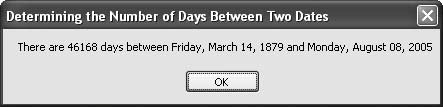# Recipe 7.14. Determining the Number of Days Between Two Dates

#### Problem

You want to calculate the number of days between two dates.

#### Solution

Sample code folder: Chapter 07\DateDiff

Use the later date's Subtract() function to calculate a TimeSpan between the two dates, and then use the Days property of the TimeSpan to get the elapsed number of days.

#### Discussion

A TimeSpan object is a representation of an elapsed amount of time. As shown in the following code, you can subtract one date from another using its Subtract() method, which returns a TimeSpan. To access the units of time from the TimeSpan, access its properties for each type of unit. For example, the following code determines the number of days a person has been on the earth by subtracting his birth date from today's date. The Days property of the resulting TimeSpan provides the desired information:

` Dim inputString As String Dim birthDay As Date Dim lifeTime As TimeSpan Dim lifeDays As Integer ' ----- Prompt the user for a date. Do    inputString = InputBox("Enter the date of your birth") Loop Until IsDate(inputString) = True ' ----- Perform the amazing calculations. birthDay = Date.Parse(inputString) lifeTime = Now.Subtract(birthDay) lifeDays = lifeTime.Days MsgBox(String.Format( _    "There are {0} days between {1:D} and {2:D}", _    lifeDays, birthDay, Now)) `

Figure 7-14 shows the number of days since Albert Einstein was born (as of August 8, 2005).

##### Figure 7-14. Determining the difference between two datesThe five span-generating members are Days, Hours, Minutes, Seconds, and Milliseconds. These each return a whole integer value stating the difference between the two dates or times. Five additional properties (TotalDays, TotalHours, TotalMinutes, TotalSeconds, and TotalMilliseconds) return decimal values that are not rounded to the nearest interval.Visual Basic 2005 Cookbook: Solutions for VB 2005 Programmers (Cookbooks (OReilly))
ISBN: 0596101775
EAN: 2147483647
Year: 2006
Pages: 400

Similar book on Amazon